# Extract Topography

This example will demonstrate how to add a cell data field to an input data set that defines whether that cell should be active. The activity of the cell is determined by whether it is beneath and input topography surface.

This filter adds a new cell data field to an input data source defining whether that cell is beneath some input topography surface.

This example demos `PVGeo.grids.ExtractTopography`

We add a cell data field to the input data set as this allows us to use a wide range of input data types. We also add this data array as it will enable users to create model discretizations within ParaView for export to external processing software that need the entire model discretization with an active cells field.

```import os

# sphinx_gallery_thumbnail_number = 6
import pyvista
from pyvista import examples

from PVGeo.grids import ExtractTopography
from PVGeo.model_build import CreateTensorMesh
```

For the grid data set, let’s use one of the Model Building sources with the following parameters:

• Origin: `[793000, 9192500, 2690]`

• X Cells: `'1000 500 50*250 500 1000'`

• Y Cells: `'1000 500 55*250 500 1000'`

• Z Cells: `'30*100.0 5*250.0 500'`

```mesh = CreateTensorMesh(
origin=[793000, 9192500, 2690],
xcellstr='1000 500 50*250 500 1000',
ycellstr='1000 500 55*250 500 1000',
zcellstr='30*100.0 5*250.0 500',
).apply()

mesh.plot(show_grid=True, color=True, show_edges=True)
```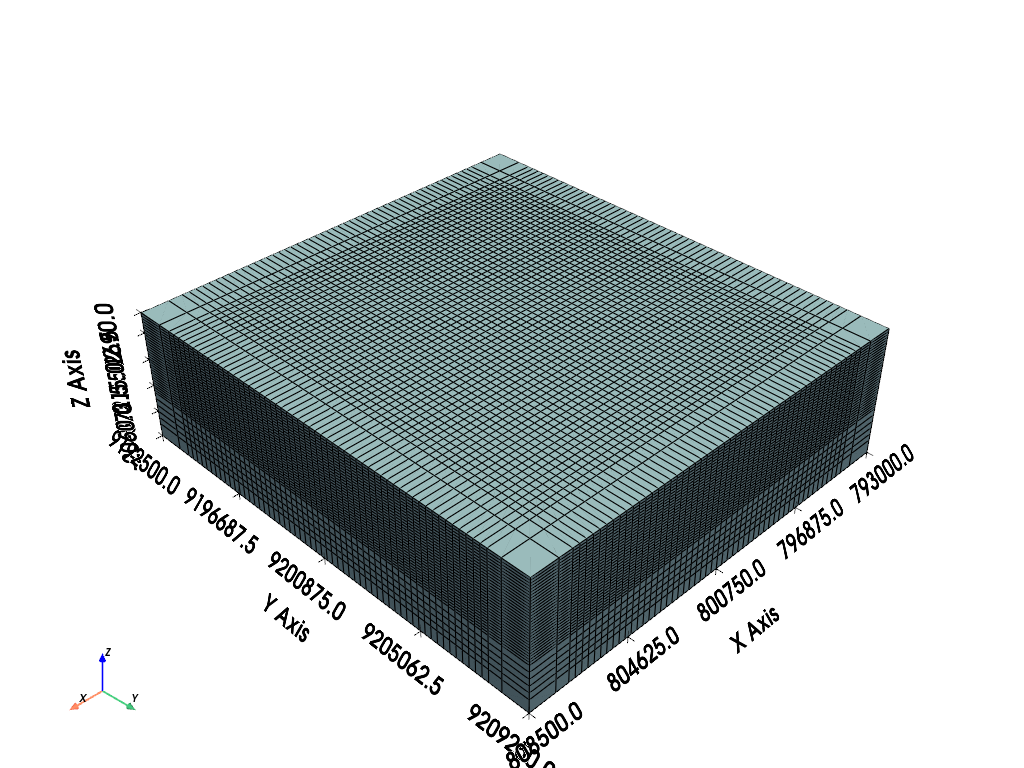Now load the topography file from the example data:

```link = 'https://dl.dropbox.com/s/gw5v3tiq68oge3l/Example-Extract-Topo.zip?dl=0'

p = pyvista.Plotter()
p.show_grid()
p.show()
```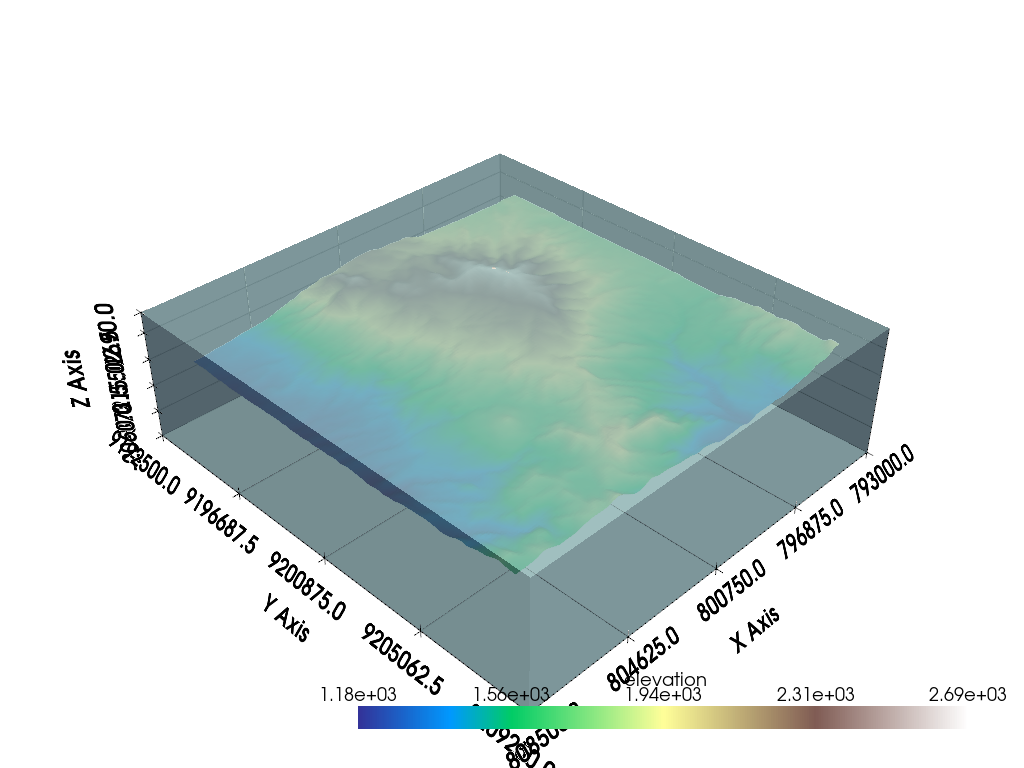Now that you have the topography and a grid data set, let’s go ahead and use the Extract Topography filter. Be sure to properly select the inputs to the algorithm.

```extracted = ExtractTopography().apply(mesh, topo)
extracted.plot(scalars='Extracted')
```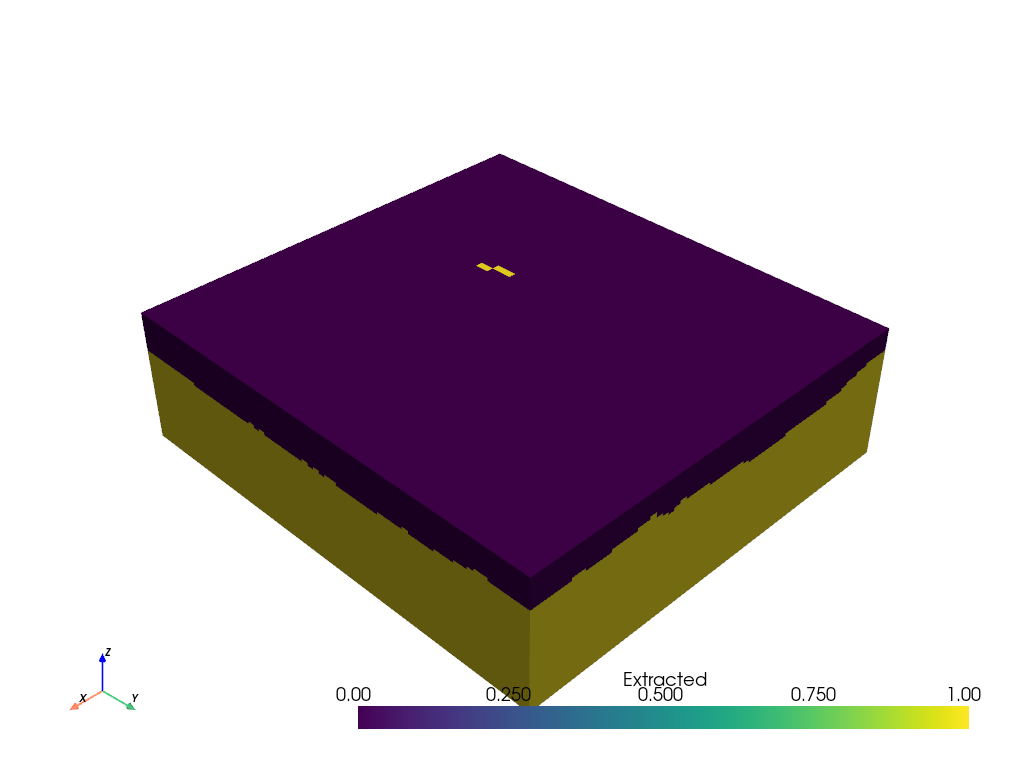op=’underneath’, tolerance=0.001, offset=0.0, invert=False, remove=False This will show the cells that are active underneath the topography surface (0 for above surface and 1 for below surface). Now we can threshold this gridded data set to remove parts of the model that are above the topography surface by applying a Threshold filter to chop out all values below 1.

The resulting grid with cells above the topography extracted will look like the rendering below:

```threshed = extracted.threshold(0.5, scalars='Extracted')
threshed.plot(color=True, show_edges=True)
```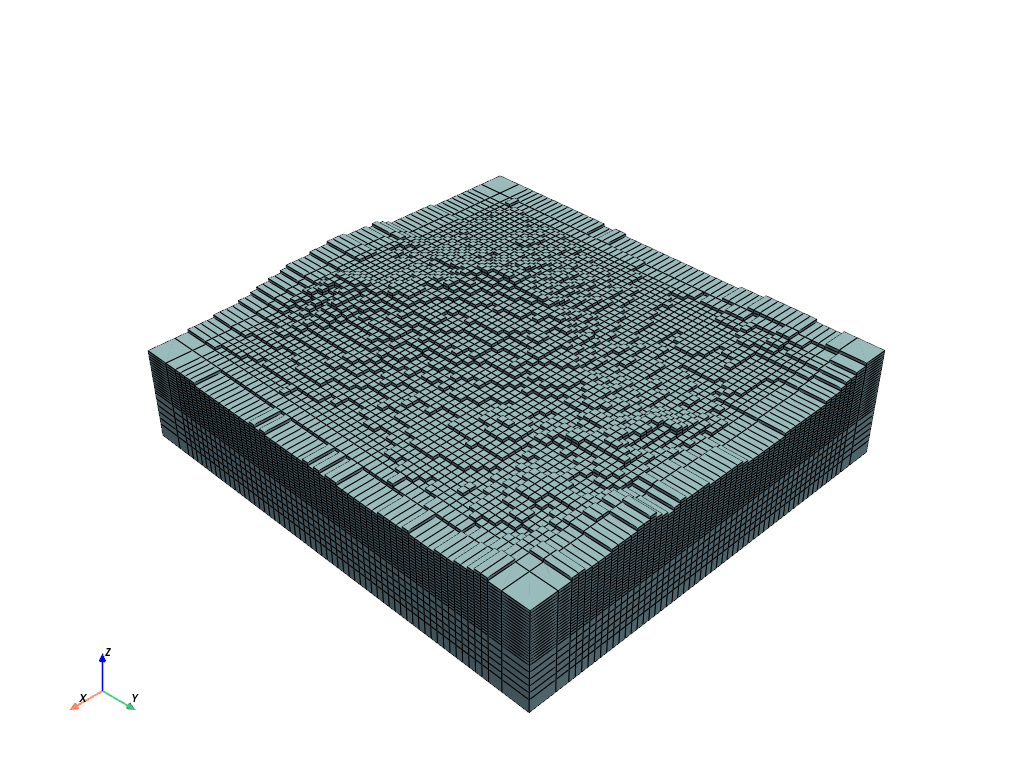How well did this remove cells above the topography surface?

```p = pyvista.Plotter()
p.show_grid()
p.show()
```Is that extraction too close to the topography surface? To better extract the topographic surface, you can set a tolerance:

```extracted = ExtractTopography(tolerance=100.0, remove=True).apply(mesh, topo)

p = pyvista.Plotter()
p.show_grid()
p.show()
```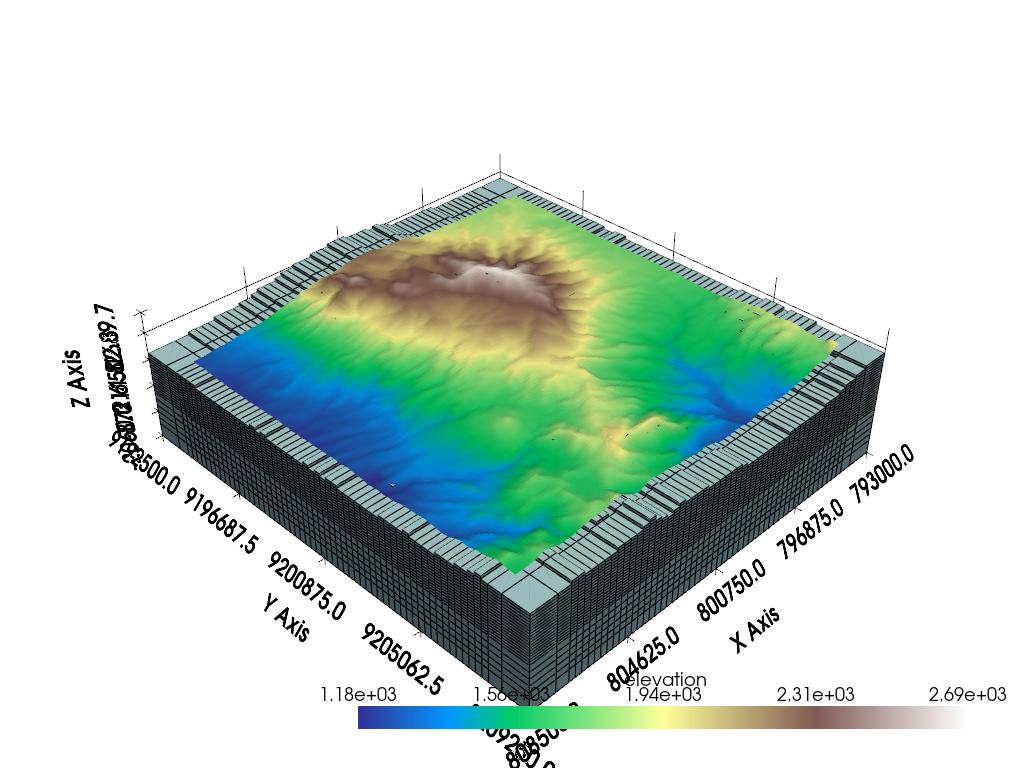Note that there are other extraction operations like an `'intersection'`:

```extracted = ExtractTopography(op='intersection', remove=True, tolerance=100.0).apply(
mesh, topo
)
extracted.plot(color=True, show_edges=True)
```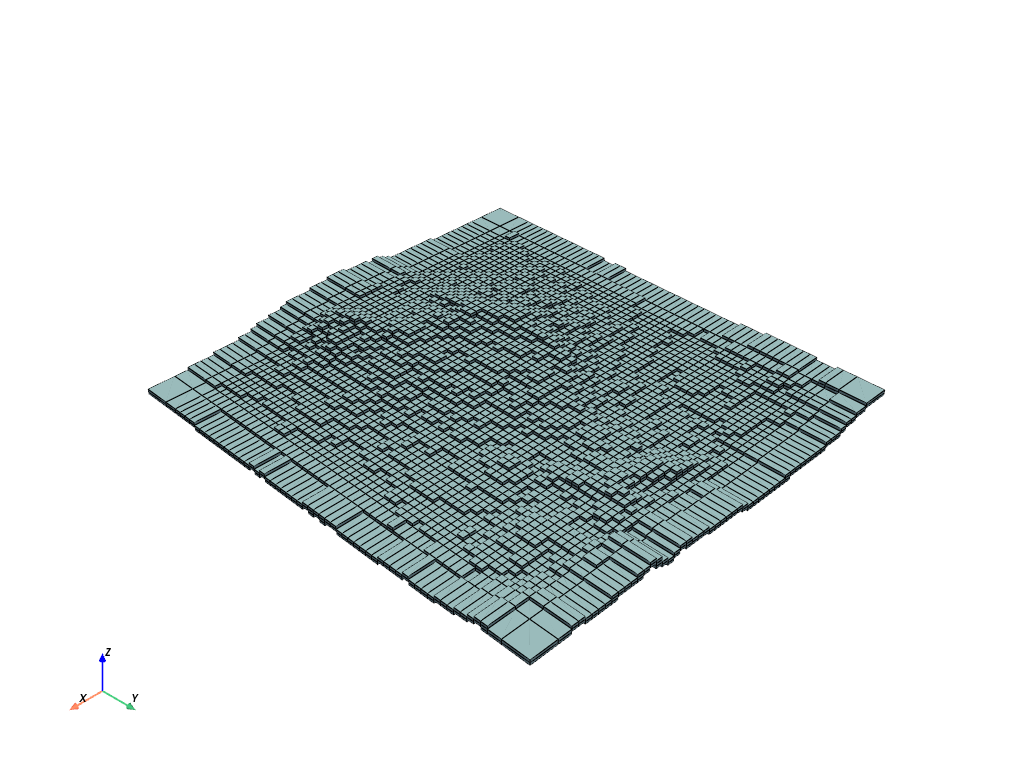Total running time of the script: ( 0 minutes 7.177 seconds)

Gallery generated by Sphinx-Gallery